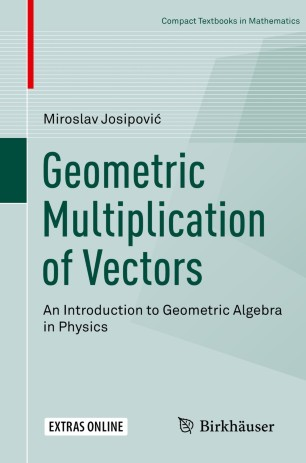# Geometric Multiplication of Vectors

## An Introduction to Geometric Algebra in Physics

• Miroslav JosipovićTextbook

Part of the Compact Textbooks in Mathematics book series (CTM)

1. Front Matter
Pages i-xxv
2. Miroslav Josipović
Pages 1-74
3. Miroslav Josipović
Pages 75-119
4. Miroslav Josipović
Pages 121-139
5. Miroslav Josipović
Pages 141-160
6. Miroslav Josipović
Pages 161-188
7. Miroslav Josipović
Pages 189-225
8. Miroslav Josipović
Pages C1-C2
9. Back Matter
Pages 227-241

### Introduction

This book enables the reader to discover elementary concepts of geometric algebra and its applications with lucid and direct explanations.

Why would one want to explore geometric algebra? What if there existed a universal mathematical language that allowed one: to make rotations in any dimension with simple formulas, to see spinors or the Pauli matrices and their products, to solve problems of the special theory of relativity in three-dimensional Euclidean space, to formulate quantum mechanics without the imaginary unit, to easily solve difficult problems of electromagnetism, to treat the Kepler problem with the formulas for a harmonic oscillator, to eliminate unintuitive matrices and tensors, to unite many branches of mathematical physics? What if it were possible to use that same framework to generalize the complex numbers or fractals to any dimension, to play with geometry on a computer, as well as to make calculations in robotics, ray-tracing and brain science? In addition, what if such a language provided a clear, geometric interpretation of mathematical objects, even for the imaginary unit in quantum mechanics?

Such a mathematical language exists and it is called geometric algebra. High school students have the potential to explore it, and undergraduate students can master it. The universality, the clear geometric interpretation, the power of generalizations to any dimension, the new insights into known theories, and the possibility of computer implementations make geometric algebra a thrilling field to unearth.

### Keywords

geometric (Clifford) algebra rotations spinors quaternions multivectors Euclidean space relativity quantum mechanics unification of mathematics universal mathematical language Pauli matrices

#### Authors and affiliations

• Miroslav Josipović
• 1
1. 1.ZagrebCroatia

Industry Sectors